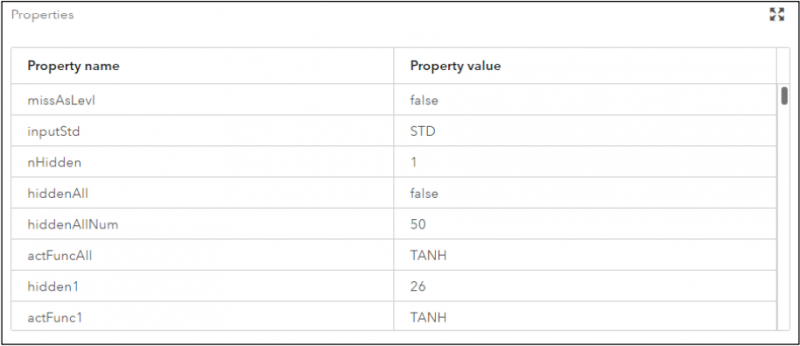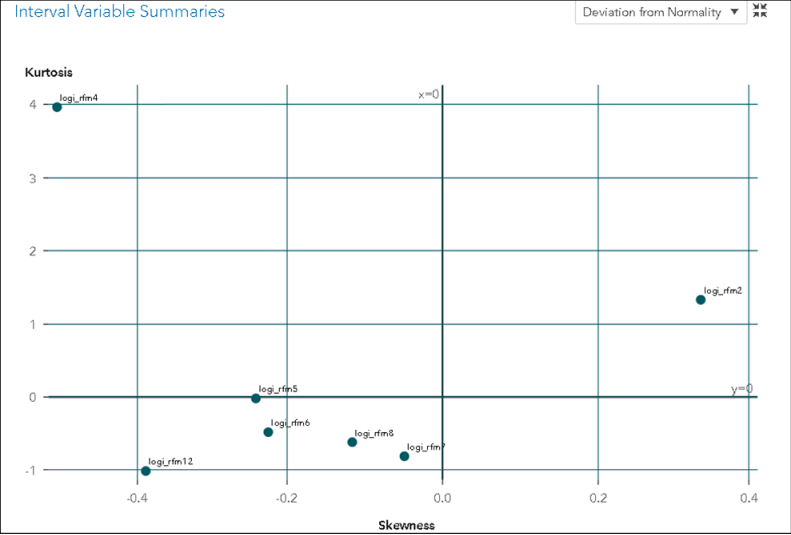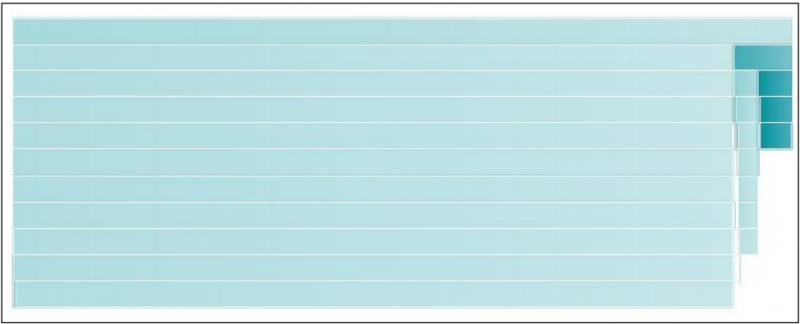# SAS Machine Learning (A00-402) Certification Exam Sample QuestionsHere are the sample questions which will help you be familiar with SAS Certified Specialist - Machine Learning Using SAS Viya 3.5 (A00-402) exam style and structure. We encourage you to try our Demo SAS Machine Learning Certification Practice Exam to measure your understanding of exam structure in an environment which simulates the SAS Machine Learning Specialist Certification test environment.

To make your preparation more easy for SAS Machine Learning Specialist (A00-402) exam, we strongly recommend you to use our Premium SAS Machine Learning Certification Practice Exam. According to our survey with certified candidates, you can easily score 85% in your actual SAS Certification exam if you can score 100% in our premium SAS Machine Learning Certification practice exams.

## SAS A00-402 Sample Questions:

01. What is another term for a feature in predictive modeling?
a) Instance
b) Input
c) Target
d) Outcome

02. Which statement is true regarding decision trees and models based on ensembles of trees?
a) In the gradient boosting algorithm, for all but the first iteration, the target is the residual from the previous decision tree model.
b) For a Forest model, the out-of-bag sample is simply the original validation data set from when the raw data partitioning took place.
c) In the Forest algorithm, each individual tree is pruned based on using minimum Average Squared Error.
d) A single decision tree will always be outperformed by a model based on an ensemble of trees.

03. Given the following properties for a neural network model, which statement is true regrading hidden units in the model? The following SAS program is submitted:a) There are no hidden units in the model
b) The number of hidden units is 1.
c) The number of hidden units is 50.
d) The number of hidden units is 26.

04. As the number of input variables in a problem increases, there is an exponential increase in the number of observations needed to densely populate the feature space. This is referred to as:
a) Problem of rare events
b) Multicollinearity
c) Curse of Dimensionality
d) Underfitting

05. Which statements are true for the F1 score?
(Choose 2.)
a) F1 score is calculated based on a depth value
b) F1 score is calculated based on a cut off value
c) F1 score is applicable to a model with a binary target.
d) F1 score is applicable to a model with an interval target.

06. Refer to the exhibit belowBased on the output from the Data Exploration node shown in the exhibit, which variable has the most thin tails (most platykurtic distribution)?
a) Logi_rfm4
b) Logi_rfm6
c) Logi_rfm8
d) Logi_rfm12

07. In Model Studio, you have multiple pipelines in a project. Which statement is true?
a) The Model Comparison node compares only the champion models for each project.
b) The Pipeline Comparison tab compares all of the models from each pipeline.
c) You can override the champion in a Model Comparison node.
d) You can override the champion in a Pipeline Comparison tab.

08. Which feature extraction method can take both interval variables and class variables as inputs?
a) Principal component analysis
b) Autoencoder
c) Singular value decomposition
d) Robust PCA

09. Refer to the treemap shown in the exhibit belowWhich statement is true about the tree map for a decision tree with a binary target?
a) The top bar represents the node with the highest probability of event
b) The darker bars represent nodes with a lower probability of event.
c) The top bar represents the node with the highest count
d) The wider bars represent nodes with a higher probability of event.

10. A project has been created and a pipeline has been run in Model Studio. Which project setting can you edit?
a) Advisor Options for missing values
b) Rules for model comparison statistic
c) Partition Data percentages
d) Event-based Sampling proportions